# Using the Physical %Complete column in EVM earned value calculations

1. In the software option and Schedule tab, change the scheduling settings to the Auto Schedule option.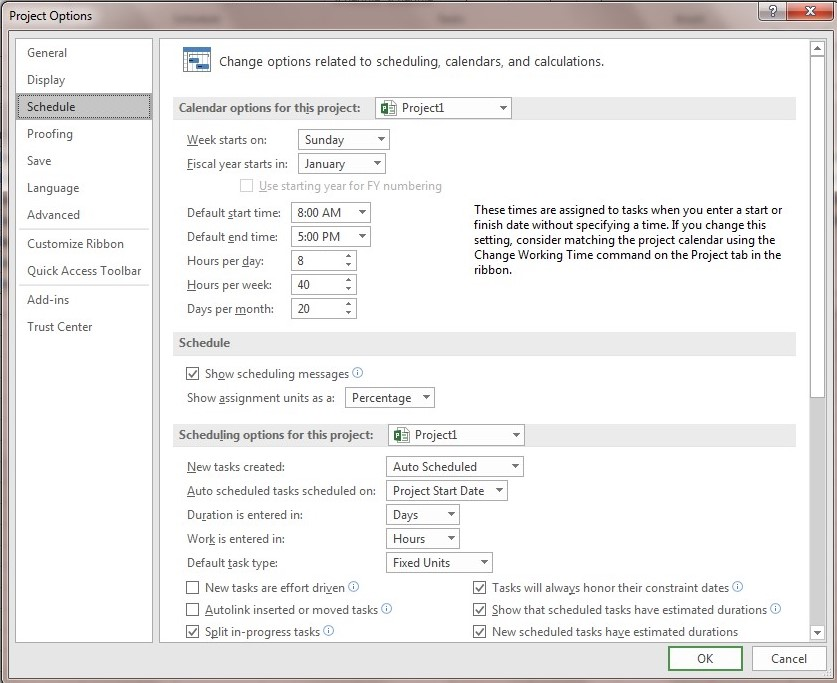1. Create a project named Sample and define the work breakdown structure, activities, duration of each activity and prerequisite relationships as follows.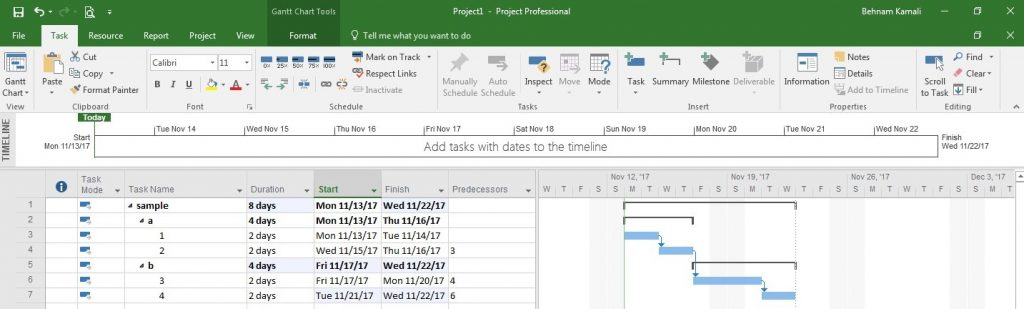1. Set the start time of the project on November 1, 2017.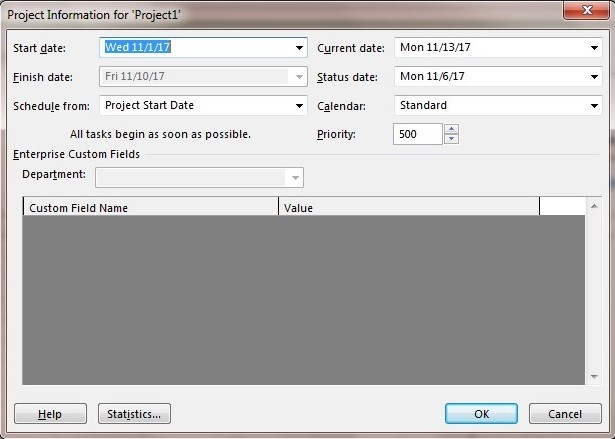4. Select Option from the File menu and make the following settings in the Advanced tab.1. Click on each activity and open the Advanced tab in the Information Task window and set the method of calculating the acquired value to Physical %Complete.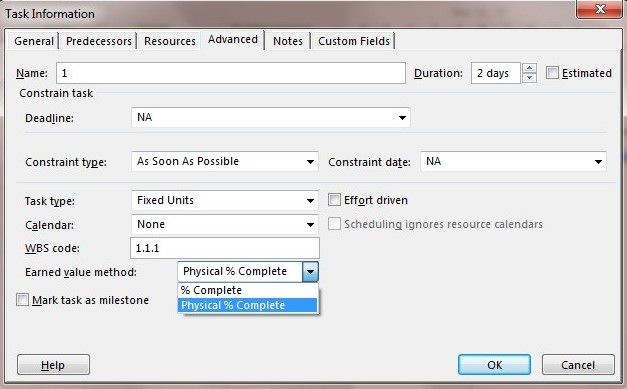1. Define sources aa and bb with the standard rate of 1000 and 2000 dollars, respectively. Both sources are work.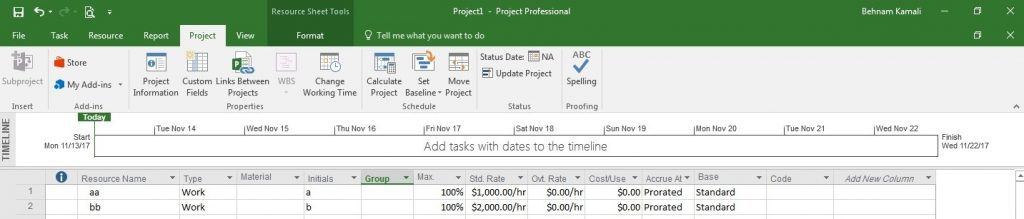1. Assign resource aa to activities 1 and 2 with 100% access and resource bb to activities 3 and 4 with 100% access.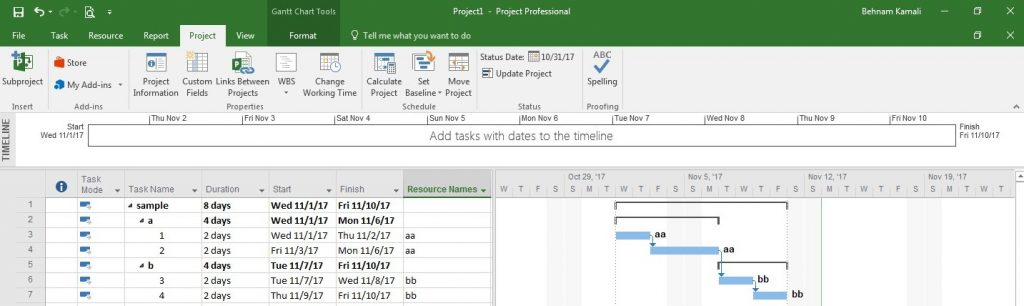1. Prepare a baseline from your project.1. Display the Baseline Cost column to display the planned cost of the project. The planned cost of the project is \$96,000.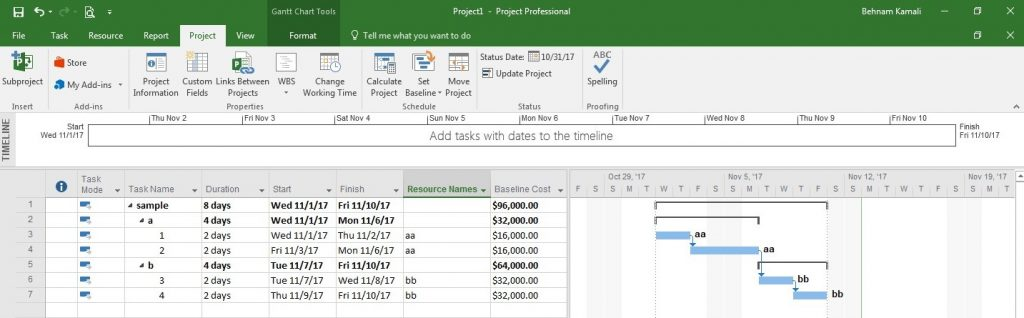1. Enter the column for Scheduled Value (BCWS), Actual Cost (ACWP) and Earned Value (BCWP).
2. Display %Complete and Physical %Complete columns. Change the project update date to the beginning of the project so that the above values ​​are changed to zero.
3. Enter the columns related to SPI, CPI, CV, SV, EAC, TCPI. In the beginning, all the mentioned columns except EAC and TCPI will show zero.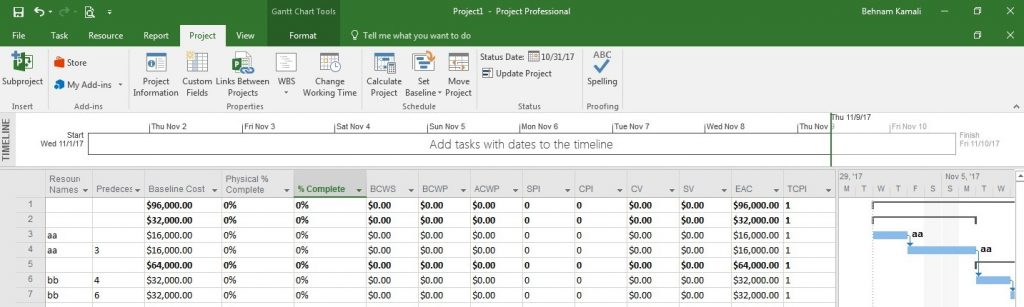1.  Enter the information related to the physical progress of the activities carried out until November 6 in the Physical %Complete column and the actual cost of each activity in the Actual Cost column according to the information below. If it is not possible to register the actual costs of the activities manually, make the following settings in order to issue a license to register the information related to the actual cost.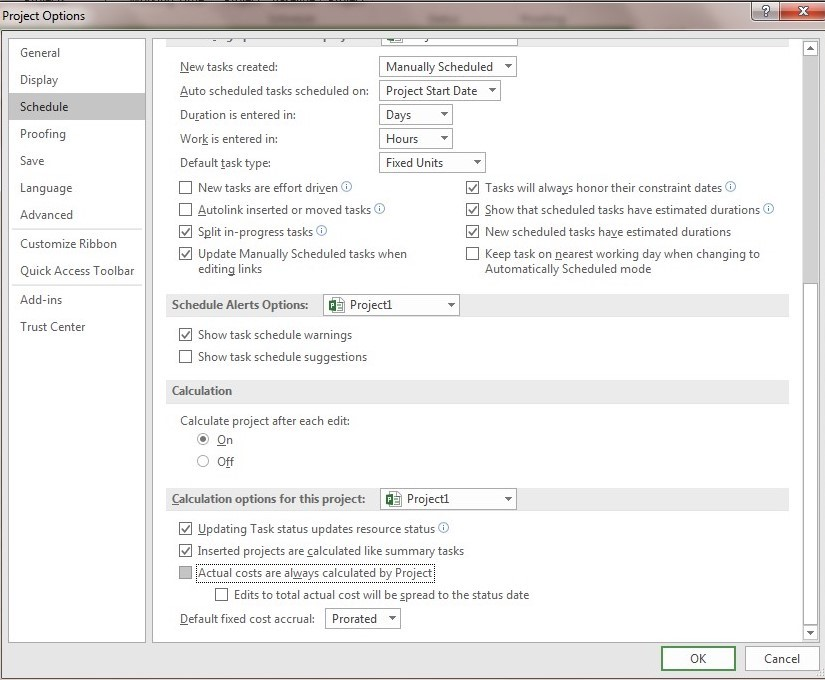The physical progress (Physical % Complete) of activity number one is 100% and the actual cost spent is \$18,000.

The physical progress (Physical % Complete) of activity number two is 100% and the actual cost spent is \$19,000.

1. Update the project on November 6. After updating, the following information is extracted: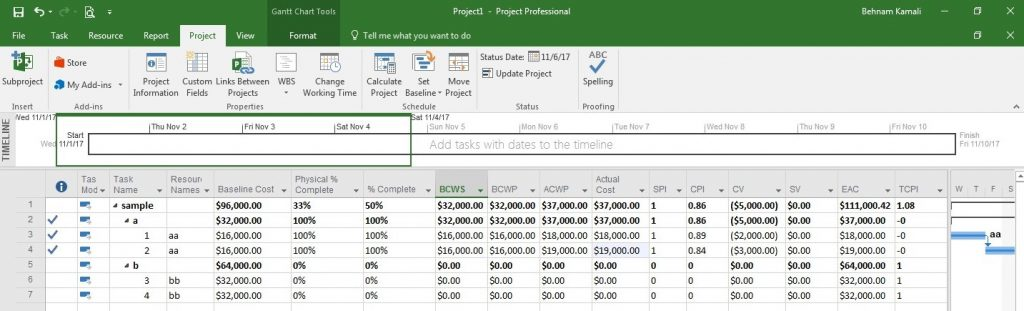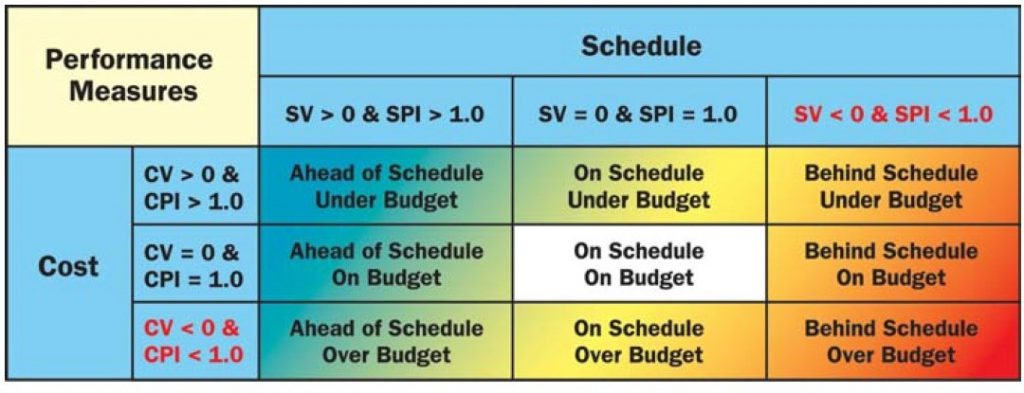Analysis of the results:

### index

The total planned cost of the project is \$96,000. This figure is created after preparing the Baseline.

96000

Base line cost

The actual physical progress rate of the entire project is 33%, which is calculated based on the actual information entered for each activity. It is worth mentioning that this figure is calculated based on the cost weight of each activity. This figure is a reliable number to present the progress of the project.

33%

Physical % complete

The progress rate of the entire project is calculated based on the status date of the project update. It is worth mentioning that this figure is calculated based on the time weight of each activity. This figure is not a reliable number to present the progress of the project.

50%

%complete

The budget cost work scheduled is up to the update date. which is calculated automatically after updating the project. (How much money should be spent until the update date?)

32000

BCWS=PV

Budget Cost Work Scheduled

(Planned Value)

The actual cost work performed is based on physical progress information. After entering the physical progress information of each activity (manually calculated in the Physical% Complete column.) What is the earned value of the work done? How much work has been done in practice?

32000

BCWP=EV

Budget Cost Work Performed

(Earned Value)

The actual cost of the performed activity is based on the costs paid by the contractor. Usually, this figure is different from the planned cost and is entered manually for each activity, and their sum is calculated as the sum of the actual cost.

37000

ACWP=AC

Actual Cost Work Scheduled

(Actual Cost)

It is calculated based on the previous field.

37000

ACTUAL COST

It is a time control index. If it’s less than one, it means we’re behind schedule. If it is equal to one, it means that we have acted according to the schedule.

1

SPI=EV/PV

(Schedule Performance Index

It is a cost control index. If it is less than one, it means that we have spent more than the approved (planned) budget for the completed works. (For every monetary unit invested, 0.86 monetary units have been earned.)

0.86

CPI=EV/AC

(Cost Performance Index)

It shows cost deviation. Less than zero means more has been spent than the plan.

-5000

CV=EV-AC

(Cost Variance)

It shows the time deviation. Zero means that the project has been carried out according to the schedule and we have no time delay.

0

SV=EV-PV

(schedule variance)

With this project cost situation, how much is the estimated cost of completing the project?

111000

EAC=BAC/CPI

(Estimate At Completion)

If we want the current project to be finished with the same planned cost (96000), what should be the productivity of the project’s resources?

1.08

TCPI

(To Complete Performance Index)### The most practical formulas of Primavera

#### گروه بندی مقالات

###### سایر مقالات تخصصی